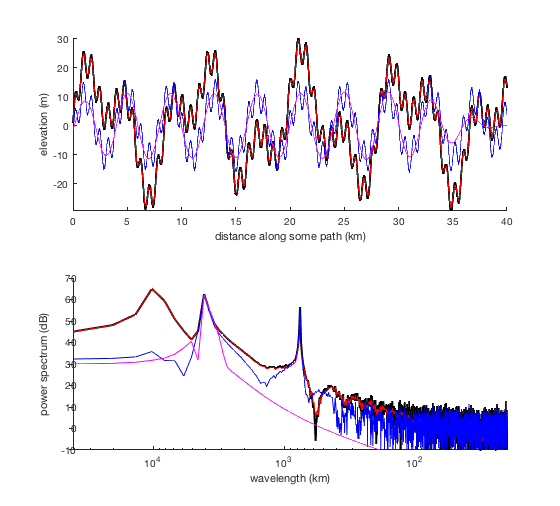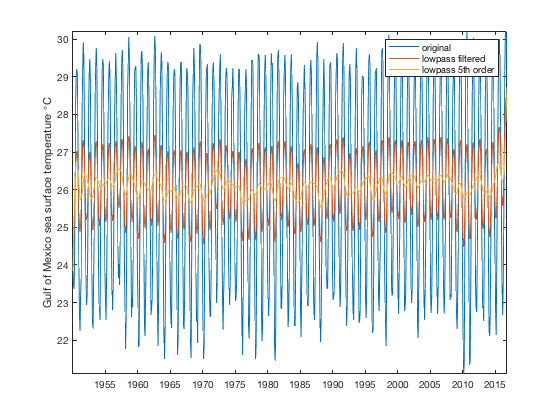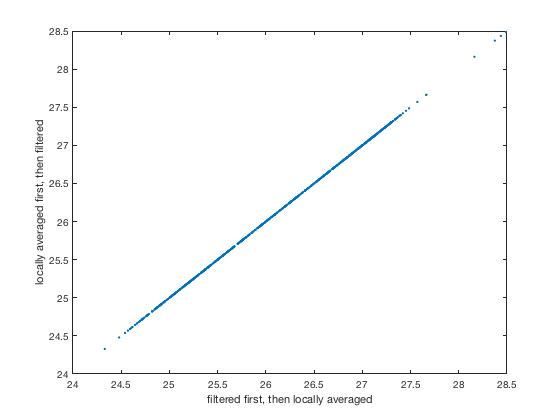# filt1 documentation

The filt1 applies a zero-phase butterworth filter. Requires Matlab's Signal Processing Toolbox.

## Syntax

```yf = filt1(filtertype,y,'fc',Fc)
yf = filt1(filtertype,y,'Tc',Tc)
yf = filt1(filtertype,y,'lambdac',lambdac)
yf = filt1(...,'fs',Fs)
yf = filt1(...,'x',x)
yf = filt1(...,'Ts',Ts)
yf = filt1(...,'order',FilterOrder)
yf = filt1(...,'dim',dim)
[yf,filtb,filta] = filt1(...)```

## Description

yf = filt1(filtertype,y,'fc',Fc) filters 1D signal y using a specified filtertype and cutoff frequency Fc. For high-pass or low-pass filters Fc must be a scalar. For band- pass and band-stop filters Fc must be a two-element array. The filtertype can be

• 'hp' high-pass with scalar cutoff frequency Fc
• 'lp' low-pass with scalar cutoff frequency Fc
• 'bp' band-pass with two-element cutoff frequencies Fc
• 'bs' band-stop with two-element cutoff frequencies Fc

yf = filt1(filtertype,y,'Tc',Tc) specifies cutoff period(s) rather than cutoff frequencies. This syntax assumes T = 1/f and is exactly the same as the 'lambdac' option, but perhaps a little more intuitive for working with time series.

yf = filt1(filtertype,y,'lambdac',lambdac) specifies cutoff wavelength(s) rather than cutoff frequencies. This syntax assumes lambda = 1/f.

yf = filt1(...,'fs',Fs) specifies a sampling frequency Fs. If neither 'fs', 'x', nor 'Ts' are specified, Fs = 1 is assumed.

yf = filt1(...,'x',x) specifies a vector of monotonically- increasing, equally-spaced sampling times or x locations corresponding to y, which is used to determine sampling frequency. If neither 'fs', 'x', nor 'Ts' are specified, Fs = 1 is assumed.

yf = filt1(...,'Ts',Ts) specifies a sampling period or sampling distance such that Fs = 1/Ts. If neither 'fs', 'x', nor 'Ts' are specified, Fs = 1 is assumed.

yf = filt1(...,'dim',dim) specifies a dimension along which to operate. By default, filt1 operates along the first nonsingleton dimension for 1D or 2D arrays, but operates down dimension 3 for 3D gridded datasets.

yf = filt1(...,'order',FilterOrder) specifies the order (sometimes called rolloff) of the Butterworth filter. If unspecified, FilterOrder = 1 is assumed.

[yf,filtb,filta] = filt1(...) also returns the filter numerator filta and denominator filtb.

## Example 1: Train Whistle

For this example we'll use the built-in train whistle example file and we'll add a little gaussian random noise to make things interesting.

```load train
y = y+0.1*randn(size(y));
```

The original signal can be plotted in black like this. First we have to build a time vector from the sampling frequency Fs:

```t = (0:length(y)-1)/Fs;

plot(t,y,'k-','linewidth',1)
box off;
axis tight
xlabel 'time (seconds)'
hold on
```If you have speakers you can listen to the train whistle like this:

`soundsc(y,Fs)`

High-pass filter the train whistle, keeping only frequencies above 750 Hz and plot it on top of the original black signal:

```yhp = filt1('hp',y,'fs',Fs,'fc',750);

hold on
plot(t,yhp,'r')
```Or perhaps you want to low-pass filter the original signal to eliminate frequencies below 1100 Hz. Note: Above we specified a sampling frequency by setting an 'fs' value. You may alternatively define a vector as the independent variable 'x'. In this case the independent variable is time, but for spatial filtering it would likely be cumulative distance along some path.

The three primary frequencies of the train whistle are spaced somewhat close together in frequency space, so the default first-order butterworth filter we used above will not eliminate all of the energy below 750 Hz. You may wish to use a steeper rolloff by specifing 'order',5. We'll plot the low-pass filtered train whistle in blue.

```ylp = filt1('lp',y,'x',t,'fc',1100,'order',5);
plot(t,ylp,'b')
```Use plotpsd to compare:

```figure
plotpsd(y,Fs,'k','linewidth',2)
hold on
plotpsd(yhp,Fs,'r')
plotpsd(ylp,Fs,'b')

xlabel 'frequency (Hz)'
axis([600 1300 0 0.02])
legend('original signal','highpass 800 Hz',...
'lowpass 1100 Hz','location','northwest')
legend boxoff
``````clear variables
close all
```

## Example 2: Topographic Profile

Suppose you have a topographic profile with elevation measurements every 10 meters for 40 kilometers. And let's say the profile has three dominant wavelengths--761 m, 4 km, and 9.4 meters. The profile might look like this. As in Example 1, I'm using plotpsd to plot a periodogram.

```SpatialRes = 10;       % Samples every 10 m
x = 0:SpatialRes:40e3; % Domain 0 to 40 km
lambda1 = 761;         % 761 meters
lambda2 = 4000;        % 4 kilometers
lambda3 = 3000*pi;     % ~9.4 kilometers

% Generate profile:
y = rand(size(x)) + 5*sin(2*pi*x/lambda1) + ...
11*sin(2*pi*x/lambda2) + 15*sin(2*pi*x/lambda3) ;

% Plot profile:
figure('position',[100 100 560 506])
subplot(211)
plot(x/1000,y,'k','linewidth',2)
hold on
xlabel 'distance along some path (km)'
ylabel 'elevation (m)'
box off
axis tight

% Plot power spectrum:
subplot(212)
plotpsd(y,x,'k','linewidth',2,'db','log','lambda')
hold on
xlabel 'wavelength (km)'
ylabel 'power spectrum (dB)'
axis tight
ylim([-10 70])
```Above you can see the three primary wavelengths as three peaks in the periodogram.

Perhaps you want to elimiate the high-frequency random noise we added to the topography with rand. To do that, you can lowpass filter out all wavelenths shorter than 300 m:

```ylo = filt1('lp',y,'x',x,'lambdac',300);

subplot(211)
plot(x/1000,ylo,'r')

subplot(212)
plotpsd(ylo,x,'r','db','log','lambda')
```Above, when we lowpass filtered the topography we specified an array x as the path distance corresponding to y. Alternatively, we could have specified spatial resolution which is our sampling distance 'Ts' to achieve the same result. Below we highpass filter the original topography to remove wavelengths longer than 6 km. Use a tight rolloff by specifying a 5th order butterworth filter.

```yhi = filt1('hp',y,'Ts',SpatialRes,'lambdac',6000,'order',5);

subplot(211)
plot(x/1000,yhi,'b')

subplot(212)
plotpsd(yhi,x,'b','db','log','lambda')
```Perhaps you want to remove high-frequency noise and the low frequencies. You can do that by filtering the signal twice, or with a bandpass filter. We can retain only that middle peak in the power spectrum by bandpass filtering out all wavelengths shorter than 3000 meters or longer than

```ybp = filt1('bp',y,'x',x,'lambdac',[3000 5000],'order',3);

subplot(211)
plot(x/1000,ybp,'m')

subplot(212)
plotpsd(ybp,x,'m','db','log','lambda')
```Perhaps you want to remove only a range of frequencies. You can do that by subtracting a bandpassed signal from the original signal a la:

```ybs = y - ybp;
```

or you can create a bandstop filter directly with using the same syntax as we did with the bandpass filter:

```ybs = filt1('bs',y,'x',x,'lambdac',[3000 5000],'order',3);

subplot(211)
plot(x/1000,ybs,'color',[.98 .45 .02])

subplot(212)
plotpsd(ybs,x,'color',[.98 .45 .02],'db','log','lambda')

legend('original','lowpass','highpass',...
'bandpass','bandstop','location','northeast')
legend boxoff
```## Example 3: Sea ice extent

Load the example time series of sea ice extent, and only use the data from 1989 toward the present, because the older data is sampled at less than daily resolution.

```load seaice_extent.mat

% Get post-1989 indices:
ind = t>datetime(1989,1,1);

% Trim the dataset to 1989-2018:
t = t(ind);
extent_N = extent_N(ind);

figure
plot(t,extent_N)
axis tight
box off
ylabel 'northern hemisphere sea ice extent (10^6 km^2)'
```Can we try to filter out the seasonal cycle? Of course the deseason function is one way to do it, but what if we do it with this butterworth filter? A bandstop filter on the seasonal cycle requires us to define the corner frequencies, so how about everything between the 6 month period and the 2 year period?

```extent_N_filt = filt1('bs',extent_N,'fs',365.25,'Tc',[0.5 2]);

hold on
plot(t,extent_N_filt,'linewidth',2)
```## Example 4: Gridded 3D time series

Suppose you're interested in SSTs in the Gulf of Mexico (GoM):

```load pacific_sst

figure
imagescn(lon,lat,mean(sst,3))
cmocean thermal % colormap
hold on

% A crude outline of the gulf of mexico:
gomlon = [-91.4 -103.8  -98.8  -88.6  -82.8  -82.3];
gomlat = [33.0   30.6   20.4   16.6   21.8   34.1];
plot(gomlon,gomlat,'ro')

text(-92.4,23.9,'Gulf of Mexico','color','red',...
'horiz','center','vert','bot') % sets text alignment
```We can use geomask and local to get a time series of GoM SSTs. First, make the mask and plot it as a blue contour just to make sure the mask is about in the right spot:

```[Lon,Lat] = meshgrid(lon,lat);

```With the mask defined, getting a 1D time series of mean SSTs in the Gulf of Mexico is easy:

```sst_gom = local(sst,mask,'omitnan');

figure
plot(t,sst_gom)
axis tight
datetick('x','keeplimits')
ylabel 'Gulf of Mexico sea surface temperature \circC'
```This dataset is at monthly temporal resolution, so if we want to lowpass filter it, keeping only frequencies with periods longer than 18 months, we could operate on the 1d sst1 dataset like this:

```% lowpass filtered 1D sst1:
sst_gom_lp = filt1('lp',sst_gom,'Tc',18);

hold on
plot(t,sst_gom_lp)

legend('original','lowpass filtered')
```You'll notice that there's still plenty of energy at the 1 year frequency. That's because the Butterworth filter is not ideal--in frequency space its shoulders are broad. To sharpen them up and more effectively cut energy at periods shorter than 18 months, increase the order of the filter:

```% lowpass filtered 1D sst1:
sst_gom_lp5 = filt1('lp',sst_gom,'Tc',18,'order',5);

plot(t,sst_gom_lp5)
legend('original',...
'lowpass filtered',...
'lowpass 5th order')
```And now you see some interannual variability that isn't too dominated by the seasonal cycle. It's probably not a great idea to be filtering away the majority of the variability in any application, but here we're just toying with the mechanics of the function, so we'll just say it's fine and move on.

But what if, instead of filtering just the 1D array, you want to filter the time series at every grid cell? The computationally inefficient way to do it would be to nest a couple of loops, going through every row and every column of the grid, all 65x55 grid cells. But that would mean doing the same operation more than 3000 times, even for this coarse grid! Fortunately, filt1 can do it much more efficiently. If since sst is a 3D matrix, filt1 already knows to operate down the third dimension, although you can always specify 'dim',3 if you want to be extra sure.

Here's how to filter the entire 3D gridded SST time series using filt1:

```sst_lp = filt1('lp',sst,'Tc',18);
```

Now we can look at the local GoM time series of the filtered 3D sst data, and it should perfectly match:

```sst_lp_gom = local(sst_lp,mask,'omitnan');

plot(t,sst_lp_gom)
legend('original',...
'lowpass filtered',...
'lowpass 5th order',...
'local after 1st order filter')
```Indeed, taking the local mean time series of the filtered SST grid gives the same results as filtering the meal local time series. Here's a direct comparison, because that plot above is getting a bit busy:

```figure
plot(sst_lp_gom,sst_gom_lp,'.')
xlabel 'filtered first, then locally averaged'
ylabel 'locally averaged first, then filtered'
```The difference between the two is just numerical noise.

## Author Info

This function is part of the Climate Data Toolbox for Matlab. The function and supporting documentation were written by Chad A. Greene of the University of Texas at Austin.